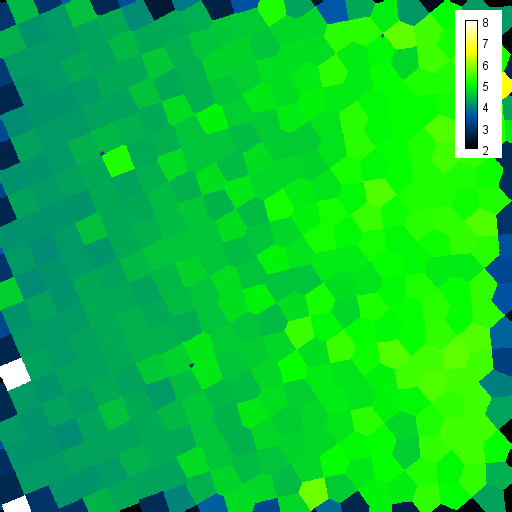# CLIJ2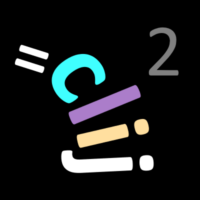GPU accelerated image processing for everyone

CLIJ2 home

# Counting neighbors in grids of cells

This notebooks introduces two techniques for counting neighbors in grids of cells.

Source

Let’s start by cleaaning up and opening an example dataset. The dataset was made by Craig S. Kaplan (@cs_kaplan) and shared via Twitter For cleaning up and loading the image, we use ImageJ-functions:

```// clean up first
run("Close All");
run("Clear Results");

// open a binary image
open("C:/structure/code/clij2-docs/md/count_neighbors/mesh_sim_from_cs_kaplan.tif");
//open("https://github.com/clij/clij2-docs/blob/master/md/count_neighbors/mesh_sim_from_cs_kaplan.tif?raw=true");
```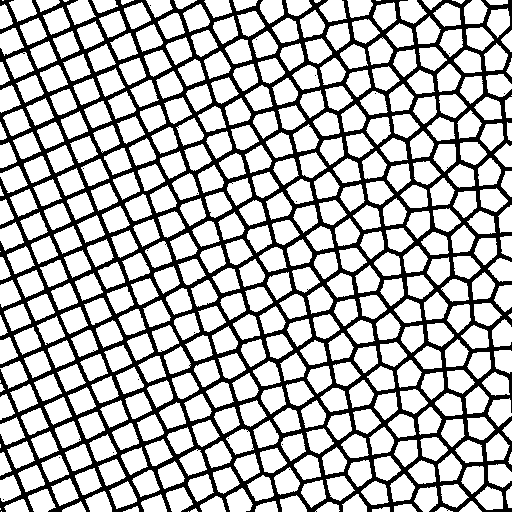We now initialize the GPU and push the image as explained in the basics of clij tutorial.

```run("CLIJ2 Macro Extensions", "cl_device=");
Ext.CLIJ2_clear();

// push image to GPU memory
input_binary = getTitle();
Ext.CLIJ2_push(input_binary);
```

# Label objects and count neighbors

We will now label the white objects in the image and generate a parametric image visualizing the number of touching neighbors similarly as explained in the section about label images and parametric images.

```Ext.CLIJ2_voronoiLabeling(input_binary, label_image);
// show label image
Ext.CLIJ2_pull(label_image);
run("glasbey_on_dark");
``````Ext.CLIJx_touchingNeighborCountMap(label_image, neighbor_count_map);
Ext.CLIJ2_pull(neighbor_count_map);
run("Green Fire Blue");
setMinAndMax(2, 8);
run("Calibration Bar...", "location=[Upper Right] fill=White label=Black number=7 decimal=0 font=12 zoom=1 overlay");
```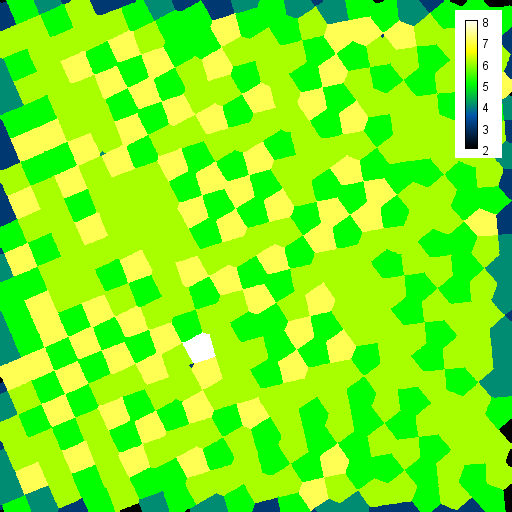Obviously, the neighbor-count sufferes from positional noise of labeled pixels with different labels. We need to try a different approach.

## Measuring touch-portion

Touch-portion describes the number of edges pixels between two labels. For example, in a perfect grid of squared labeled objects, as shown on the left, all labels touch by 0.25, or 25%. If a cell has five neighbors, touch-portion is 0.2 or 20%. We can visualize touch-portion as a mesh between label centroids:

```Ext.CLIJx_drawTouchPortionMeshBetweenTouchingLabels(label_image, touch_portion_mesh);
Ext.CLIJ2_pull(touch_portion_mesh);
run("Green Fire Blue");
setMinAndMax(0, 1);
run("Calibration Bar...", "location=[Upper Right] fill=White label=Black number=5 decimal=2 font=12 zoom=1 overlay");
```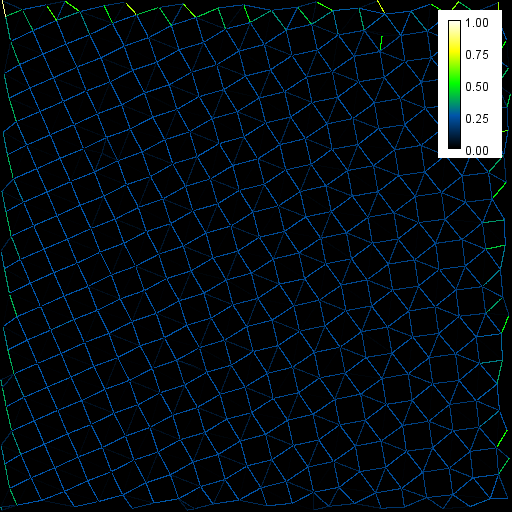By changing brightness, contrast and the lookup-table we can highlight differences between the left and the right region:

```run("Duplicate...", "title=copy");
run("Rainbow RGB");
setMinAndMax(0.1, 0.3);
run("Calibration Bar...", "location=[Upper Right] fill=White label=Black number=3 decimal=2 font=12 zoom=1 overlay");
```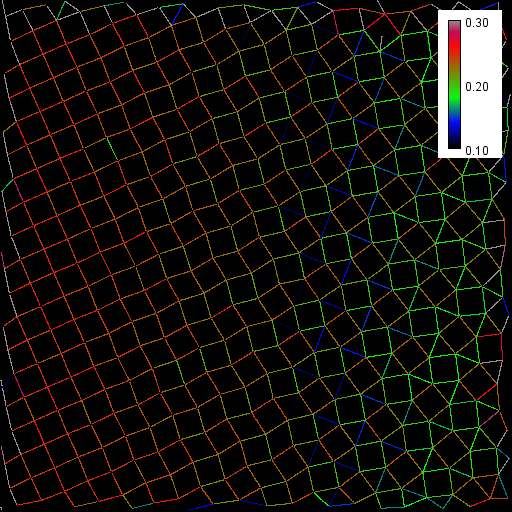The median touch-portion per labeled object gives us an impression of the distribution in space:

```Ext.CLIJx_medianTouchPortionMap(label_image, median_touch_portion_map);
Ext.CLIJ2_pull(median_touch_portion_map);
run("Rainbow RGB");
setMinAndMax(0.1, 0.3);
run("Calibration Bar...", "location=[Upper Right] fill=White label=Black number=3 decimal=2 font=12 zoom=1 overlay");
```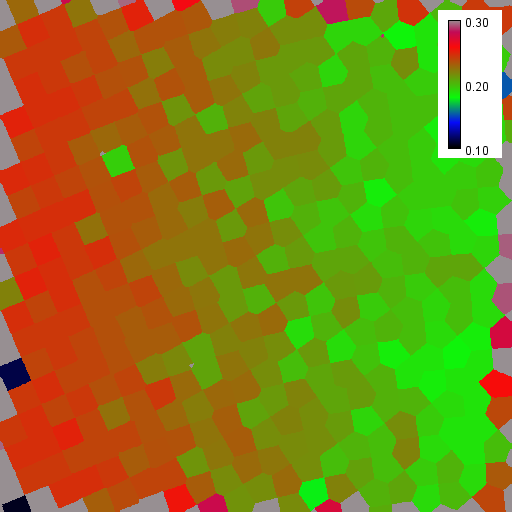This map can be used to draw a map of estimated, continous neighbor-count. We know labels in a symmetric grid with 4 neighbors have a median touch-portion of 0.25, and if they have 5 neighbors, median touch-portion is typicall 0.2. Thus, we can estimate neighbor_count = 1 / median_touch_portion:

```Ext.CLIJx_divideScalarByImage(median_touch_portion_map, estimated_neighbor_count, 1.0);
Ext.CLIJ2_pull(estimated_neighbor_count);
run("Green Fire Blue");
setMinAndMax(2, 8);
run("Calibration Bar...", "location=[Upper Right] fill=White label=Black number=7 decimal=0 font=12 zoom=1 overlay");
```